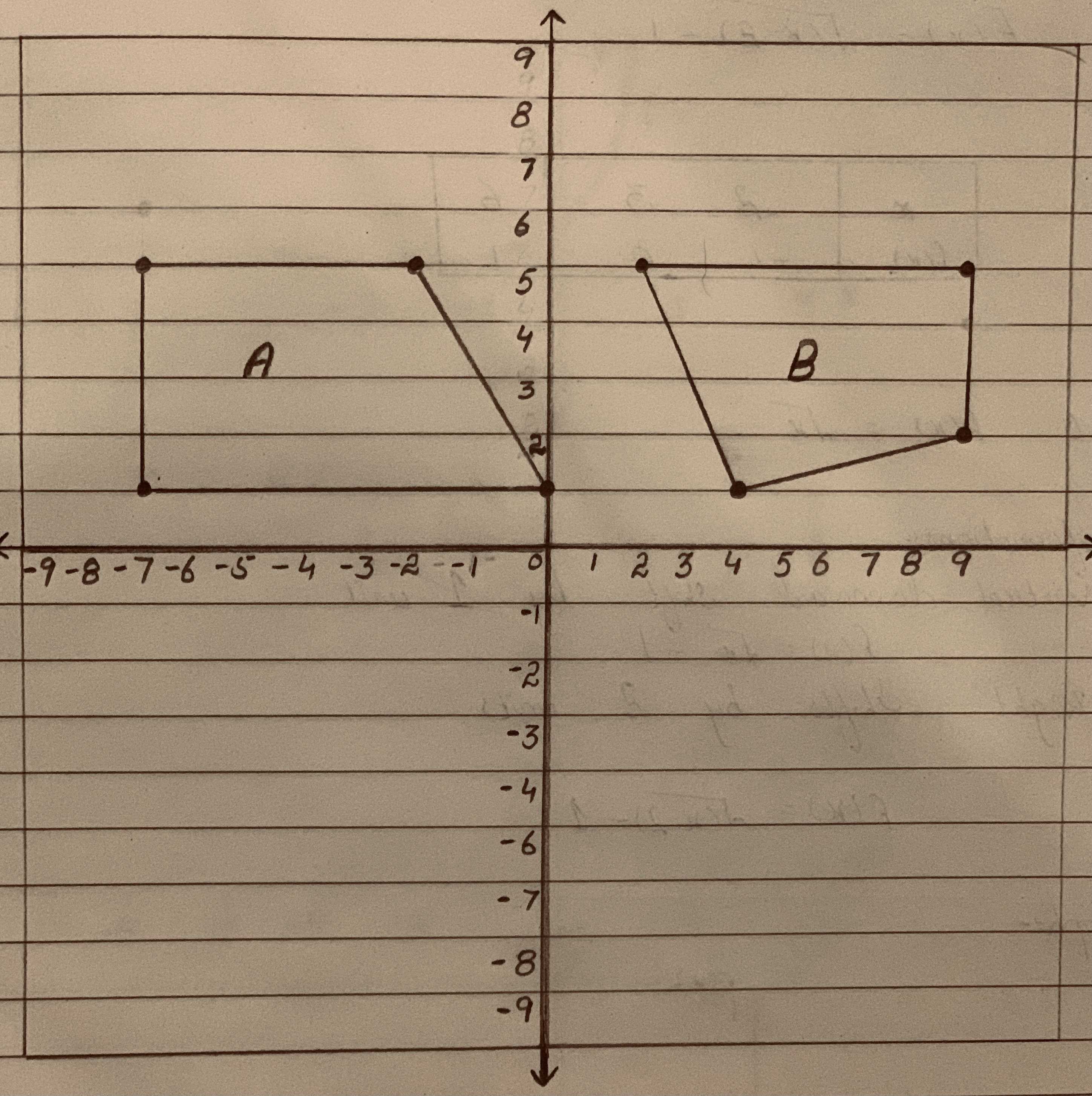# Lily wants to define a transformation (or series of transformations) using only rotations, reflections or translations that takes Figure A to Figure BbanganX 2021-02-03 Answered

Lily wants to define a transformation (or series of transformations) using only rotations, reflections or translations that takes Figure A to Figure B.Which statement about the transformation that Lily wants to define is true?
A. It can be defined with two reflections.
B.It can be defined with one rotation and one translation.
C. It cannot be defined because Figure A and Figure B are not congruent.
D.It cannot be defined because the longest side of Figure B is on the bottom.

You can still ask an expert for help

• Questions are typically answered in as fast as 30 minutes

Solve your problem for the price of one coffee

• Math expert for every subject
• Pay only if we can solve ityagombyeR
Observe that the figure A is a quadrilateral with no sides parallel.
The figure B is a quadrilateral with a pair of opposite sides parallel. That is figure B is a trapezium.
The figure A and figure B are not congruent, since A is a quadrilateral and B a trapezium.
And thus, Figure A cannot be transformed to figure B by using only rotations, reflections or translations.
The correct option is C.
It cannot be defined because Figure A and Figure B are congruent.